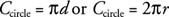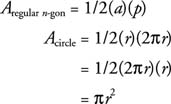## Circles

circle is a planar figure with all points the same distance from a fixed point. That fixed point is called the center of the circle. Any segment that goes from the center to a point on the circle is called a radius of the circle. A diameter is any segment that passes through the center and has its endpoints on the circle. Obviously, a diameter is twice as long as a radius. In Figure 1O is the center, OB OC , and OA each a radius, and ACis a diameter.Figure 1 A circle with center, radius, and diameter labeled.

## Finding the Circumference

In ancient times the Greeks discovered that for all circles, the circumference divided by the diameter always turns out to be the same constant value. The Greek letter π (pi) is now used to represent that value. In fractional or decimal form, the commonly used approximations of π are π ≈ 3.14 or π ≈ 22/7. The Greeks found the formula Ccircle/d = π, which is rewritten in the following form.If you briefly regard a circle as a regular polygon with infinitely many infinitesimally small sides, you see that the apothem and radius become the same length (Figure 2).Figure 2 Apothem and radius of a circle.

Looking at the area formula for a regular polygon and making the appropriate changes with regard to the circle,That is, the formula for the area of a circle now becomes the following:Example 1: Find the circumference and area for the circle in Figure 3. Use 3.14 as an approximation for π.Figure 3 Finding the circumference and area of a circle.Example 2: If the area of a circle is 81π ft2, find its circumference.So the circumference is approximately 56.52 ft.